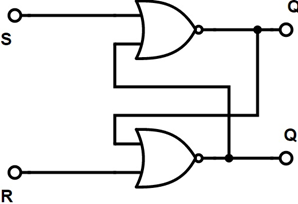# FLIP FLOP AS A FREQUENCY DIVIDER

``````                              FLIP FLOP
``````

The flip flop is an electronic circuit which is used to store data which has two stable states. Binary data are stored by this and we can change the data by applying varying inputs. Latches and flip flops are the main building blocks of digital electronic systems used in computers, mainly both flip flop and latches are used for data storage. Flip flop is a bistable multivibrator the circuits can be changed by applying signals to one or more control inputs and will have one or two outputs flip flop is a device which stores single bit of data it has two states one of it represents “one” and other represents “zero” these kinds of storage and such a circuit is described as sequential logic

D FLIP FLOP

In flip, flop the output not only depends on the present inputs but also depends in the former inputs and outputs. The difference between a flip flop and latch circuit is a latch doesn’t use a clock signal while flip flop does. Flip flop circuit designing is done by using logic gates such as two NAND and NOR gates

TYPES OF FLIP FLOP SR FLIP FLOP It is built with 2 and gates and a NOR flip flop if the clock pulse is zero the outputs of the two AND gates will remain zero. When the clock pulse is one the information of S and R will pass through Flip flop when S=R=1 by the clock pulse both the outputs will be zero the state of flip flop unsated when the clock pulse is detachedD FLIP FLOP The simplification of SR flip flop is D flip flop it is known as “data” or “delay” flip flop the value of D input is captured by the D flip flop during a certain portion of clock cycle and the captured value will become Q outputs at other time Q output doesn’t change

JK FLIP FLOP It is an improvement in SR flip flop were S=R=1 is not a problem due to this condition J=k=1 the output state will be invertedFREQUENCY DIVISION BY FLIP FLOP It can be used as a binary divider for frequency division by using toggle flip flop we can do frequency division with a small modification of JK flip flop we can create a new type of flip flop called toggle flip flop toggle flip flop can be made from D type flip flop or standard JK flip flop the toggle flip flop has got its name by its ability to toggle or switch between its two different states the “memory” and “toggle” states. Ripple binary counters can be created by using toggle flip flop it could build ripple counters as it toggles from one state to another

APPLICATIONS • Shift registers • Frequency divider • Counters • Storage registers • Data storage • Data transfer • Latch